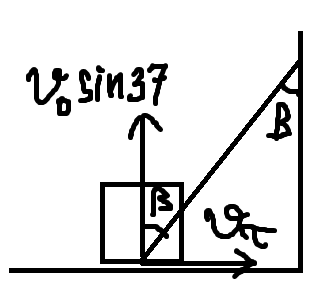# A block moving on the floor and hitting the walls

Do we have to keep this is mind for the second collision as well?

Why not :). But after calculating the second reflection angle, you may get negative value of angle. Thus the behaviour of the second collision is quite different from the first one. Just think of it in the physics sense and give an explanation of how the block should reflect after the second collision

1 симпатия

I have got this equation for reflection angle from second collision. I have taken beta as the refle tion angle from wall 2.

this equation is essentially the same as what i did under wrong assumption that the speed doesn’t change. use my another tip Help in this question - #36 от пользователя Alisher

1 симпатия

I am not able to get the answer.

when expressing the conservation of momentum along the wall, you have to write

\mu \int Ndt = mv_0(\cos37-v_\tau) = \mu\cdot 2mv_0\sin37 \Rightarrow \\ v_\tau = v_0(\cos37 - 2\mu\sin37).here I denote \beta in the way I drew above, i.e. \tan\beta=v_\tau/v_0\sin37 = \cot44.82 - 2\mu_1, so \beta = 44.82\degree. Also,

v_1 =\sqrt{(v_0\sin37)^2+v_\tau^2}=\frac{v_0\sin37}{\cos\beta}= 8.48 \space \text{m/s}.

Then I use the same thing to calculate second reflection angle (remember it’s with respect to the normal line!):

\gamma = \arctan(\cot44.82 - 2\mu_2) = -26.26\degree.

Well, the \gamma<0 thing does not sound very physical; the result implies that the block has changed its direction. But the main catch is that friction force \mu N occurs only when relative movement occurs, so apparently during this infinitesimally short interval of time, the block has stopped moving relative to the second wall. Thus, the real reflection angle is 0, i.e. perpendicular to the second wall. Therefore, we get

v_2 = v_1\sin44.82 = 5.98\space\text{m/s}.
3 симпатии

Oh my god… finally I understand the whole problem… Thanks a lot!

1 симпатия

One more doubt, why did you take the sin component of V1 in the last part?

because modulus of perpendicular component of the velocity is conserved, and 44.8° is counted from the wall’s face, so i used sine

1 симпатия

Ohh, and one more thing, in the first line you used cos37 - V(parallel to first wall) is this correct ? How ?

yes, because v_\tau and v_0\cos37 are in the same direction, but the friction slows down this velocity component

1 симпатия

But you have taken out V0, and inside bracket you are still having v (parallel) ? How is this correct?

I think costheta should also have V0 written beside it…

1 симпатия

ah, i see. there should be v_\tau/v_0, but it doesn’t change the solution

1 симпатия

yeah, this is what i meant

1 симпатия

Ok. Thank you!

Ok, I’ll keep that in mind. Actually, I thought maybe long titles won’t look nice